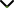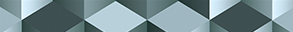# Recent Advances in Function Spaces and its Applications in Fractional Differential Equations 2020

Publishing date
01 Nov 2020
Status
Closed
03 Jul 2020

Guest Editors

1Curtin University of Technology, Perth, Australia

2Qufu Normal University, Qufu, China

3Curtin University, Perth, Australia

4Yantail University, Yantai, China

This issue is now closed for submissions.
More articles will be published in the near future.

# Recent Advances in Function Spaces and its Applications in Fractional Differential Equations 2020

This issue is now closed for submissions.
More articles will be published in the near future.

## DescriptionFractional differential equations are a new research area of analytical mathematics, which provides useful tools to model many physical and biological phenomena and optimal control of complex processes with memory effects. Function space theory has played an important role in the study of various fractional differential equations and complex real-world problems.

Therefore, by using function space theory, understanding the characteristics of solutions and developing the properties of approximate solutions of this type of equations would have a profound impact on many disciplines. The new advancements of function space theory will greatly promote the development of fractional calculus theory, functional theory, and mathematical physics, as well as their applications in differential and integral equations.

The aim of this Special Issue is to report and promote the latest achievements and recent developments in the well-posedness analysis and computational methods and function space theory for solving various fractional differential equations. We invite researchers to submit original research articles as well as review articles on the recent development in the theory of function spaces and the applications of nonlinear fractional differential equations in sciences, technologies and engineering.

Potential topics include but are not limited to the following:

• Function space theory including fractional derivative
• Boundary value problems of fractional differential equations
• Initial value problems of fractional differential equations
• Inequalities of fractional integrals and fractional derivatives
• Singular and impulsive fractional differential and integral equations
• Analysis and control in fractional differential equations
• Numerical analysis and algorithm for fractional differential equations
• Fixed point theory and application in fractional calculus
• Operator theory in function spaces and operator algebras
• Fractional functional equations in function spacesJournal metrics
See full report
Acceptance rate33%
Submission to final decision52 days
Acceptance to publication26 days
CiteScore2.400
Journal Citation Indicator1.150
Impact Factor1.281Author guidelinesEditorial boardDatabases and indexing

Article of the Year Award: Outstanding research contributions of 2021, as selected by our Chief Editors. Read the winning articles.